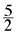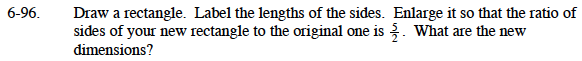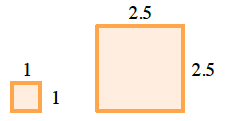### Home > MC1 > Chapter 6 > Lesson 6.2.5 > Problem6-96

6-96.

. Draw a rectangle. Label the lengths of the sides. Enlarge it so that the ratio of sides of your new rectangle to the original one is. What are the new dimensions? Homework Help ✎William was asked to draw two squares with the ratio of sides 2:5.
As you can see, each side of the original square is 1 unit, and each side of the new square is 2.5 units.
Can you find a way that William may have enlarged his squares?$\text{William multiplied each side by 2.5, or }\frac{5}{2},\text{ in order to enlarge his square.}$

Do you think this will work for your rectangles? Make sure your drawings are neat!